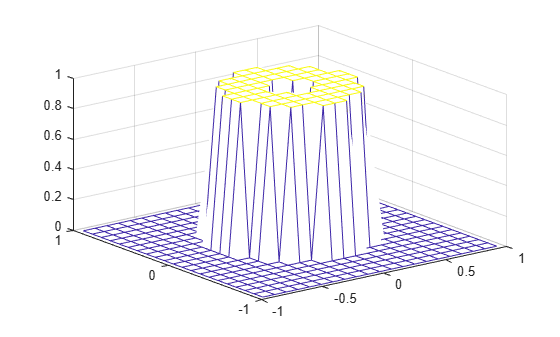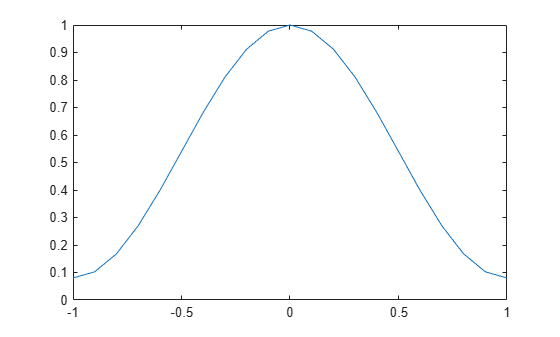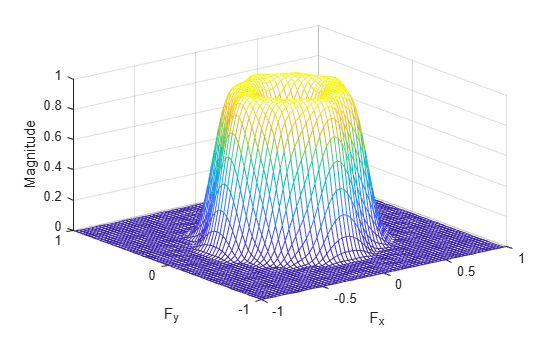# fwind1

2-D FIR filter using 1-D window method

## Syntax

``h = fwind1(Hd,win)``
``h = fwind1(Hd,win1,win2)``
``h = fwind1(f1,f2,Hd,___)``

## Description

The `fwind1` function designs 2-D FIR filters using the window method. `fwind1` uses a 1-D window specification to design a 2-D FIR filter based on the desired frequency response. `fwind1` works with 1-D windows only. Use `fwind2` to work with 2-D windows.

You can apply the 2-D FIR filter to images by using the `filter2` function.

example

````h = fwind1(Hd,win)` creates a 2-D FIR filter `h` based on the desired frequency response `Hd`. The `fwind1` function uses the 1-D window `win` to form an approximately circularly symmetric 2-D window using Huang's method.```
````h = fwind1(Hd,win1,win2)` uses two 1-D windows, `win1` and `win2`, to create a separable 2-D window.```
````h = fwind1(f1,f2,Hd,___)` enables you to specify the desired frequency response `Hd` at arbitrary frequencies `f1` and `f2` along the x- and y-axes.```

## Examples

collapse all

This example shows how to design an approximately circularly symmetric two-dimensional bandpass filter using a 1-D window method.

Create the frequency range vectors `f1` and `f2` using `freqspace`. These vectors have length 21.

`[f1,f2] = freqspace(21,'meshgrid');`

Compute the distance of each position from the center frequency.

`r = sqrt(f1.^2 + f2.^2);`

Create a matrix `Hd` that contains the desired bandpass response. In this example, the desired passband is between 0.1 and 0.5 (normalized frequency, where 1.0 corresponds to half the sampling frequency, or $\pi$ radians).

```Hd = ones(21); Hd((r<0.1)|(r>0.5)) = 0;```

Display the ideal bandpass response.

```colormap(parula(64)) mesh(f1,f2,Hd)```Design the 1-D window. This example uses a Hamming window of length 21.

`win = 0.54 - 0.46*cos(2*pi*(0:20)/20);`

Plot the 1-D window.

```figure plot(linspace(-1,1,21),win);```Using the 1-D window, design the filter that best produces this frequency response

`h = fwind1(Hd,win);`

Display the actual frequency response of this filter.

`freqz2(h)`## Input Arguments

collapse all

Desired frequency response, specified as a numeric matrix. `Hd` is sampled at equally spaced points between -1.0 and 1.0 (in normalized frequency, where 1.0 corresponds to half the sampling frequency, or π radians) along the x and y frequency axes. For accurate results, create `Hd` by using the `freqspace` function.

Data Types: `single` | `double` | `int8` | `int16` | `int32` | `int64` | `uint8` | `uint16` | `uint32` | `uint64`

1-D window, specified as a numeric matrix. If you have Signal Processing Toolbox™ software, then you can specify `win` using windows such as `hamming` (Signal Processing Toolbox), `hann` (Signal Processing Toolbox), `bartlett` (Signal Processing Toolbox), `blackman` (Signal Processing Toolbox), `kaiser` (Signal Processing Toolbox), or `chebwin` (Signal Processing Toolbox).

Data Types: `single` | `double`

1-D window, specified as a numeric matrix.

Data Types: `single` | `double`

1-D window, specified as a numeric matrix.

Data Types: `single` | `double`

Desired frequency along the x-axis. The frequency vector should be in the range [-1, 1], where 1.0 corresponds to half the sampling frequency, or π radians.

Data Types: `single` | `double`

Desired frequency along the y-axis. The frequency vector should be in the range [-1, 1], where 1.0 corresponds to half the sampling frequency, or π radians.

Data Types: `single` | `double`

## Output Arguments

collapse all

2-D FIR filter, returned as a numeric matrix. The length of the window controls the size of the resulting filter.

• If you specify a single window `win` of length `n`, then the size of `h` is `n`-by-`n`.

• If you specify two windows `win1` and `win2` of length `n` and `m` respectively, then the size of `h` is `m`-by-`n`.

If `Hd` is of data type `single`, then `h` is of data type `single`. Otherwise, `h` is of data type `double`.

Data Types: `single` | `double`

## Algorithms

The `fwind1` function takes a one-dimensional window specification and forms an approximately circularly symmetric two-dimensional window using Huang's method,

`$w\left({n}_{1},{n}_{2}\right)={w\left(t\right)|}_{t=\sqrt{{n}_{{}_{1}}^{2}+{n}_{2}^{2}}},$`

where w(t) is the one-dimensional window and w(n1,n2) is the resulting two-dimensional window.

Given two windows, the `fwind1` function forms a separable two-dimensional window:

`$w\left({n}_{1},{n}_{2}\right)={w}_{1}\left({n}_{1}\right){w}_{2}\left({n}_{2}\right).$`

The `fwind1` function calls the `fwind2` with the desired frequency response `Hd` and the two-dimensional window. The `fwind2` function calculates `h` using an inverse Fourier transform and multiplication by the two-dimensional window:

`${h}_{d}\left({n}_{1},{n}_{2}\right)=\frac{1}{{\left(2\pi \right)}^{2}}{\int }_{-\pi }^{\pi }{\int }_{-\pi }^{\pi }{H}_{d}\left({\omega }_{1},{\omega }_{2}\right){e}^{j{\omega }_{1}{n}_{1}}{e}^{j{\omega }_{2}{n}_{2}}d{\omega }_{1}d{\omega }_{2}$`
`$h\left({n}_{1},{n}_{2}\right)={h}_{d}\left({n}_{1},{n}_{2}\right)w\left({n}_{1},{n}_{2}\right)$`

 Lim, Jae S., Two-Dimensional Signal and Image Processing, Englewood Cliffs, NJ, Prentice Hall, 1990.